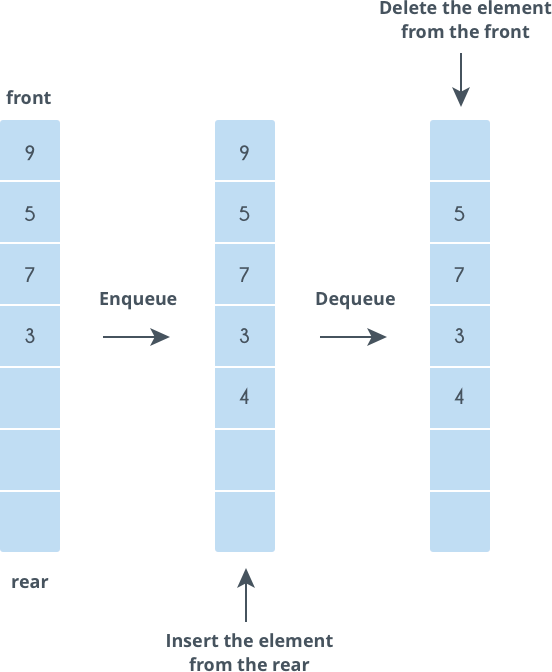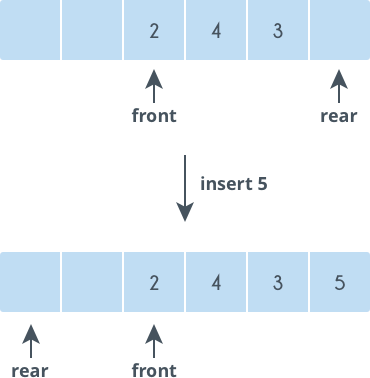Data Structures
Topics:
Basics of Queues
• Arrays
• Stacks
• Queues
• Basics of Queues
• Hash Tables
• Trees
• Advanced Data Structures
• Disjoint Data Structures

# Basics of Queues

Queues are data structures that follow the First In First Out (FIFO) i.e. the first element that is added to the queue is the first one to be removed.

Elements are always added to the back and removed from the front. Think of it as a line of people waiting for a bus. The person who is at the beginning of the line is the first one to enter the bus.

Variables used

• $queue[]$: Array in which queue is simulated
• $arraySize$: Maximum number of elements that can be stored in a $queue[]$
• $front$: Points at the index where the next deletion will be performed
• $rear$: Points at the index where the next insertion will be performed

Functions supported

Queues support the following fundamental functions:

Enqueue

If the queue is not full, this function adds an element to the back of the queue, else it prints “OverFlow”.

void enqueue(int queue[], int element, int& rear, int arraySize) {
if(rear == arraySize)            // Queue is full
printf(“OverFlow\n”);
else{
queue[rear] = element;    // Add the element to the back
rear++;
}
}


Dequeue

If the queue is not empty, this function removes the element from the front of the queue, else it prints “UnderFlow”.

void dequeue(int queue[], int& front, int rear) {
if(front == rear)            // Queue is empty
printf(“UnderFlow\n”);
else {
queue[front] = 0;        // Delete the front element
front++;
}
}


Front

This function returns the front element of the queue.

int Front(int queue[], int front) {
return queue[front];
}


Support functions

Size

This function returns the size of a queue or the number of elements in a queue.

int size(int front, int rear) {
return (rear - front);
}


IsEmpty

If a queue is empty, this function returns 'true', else it returns 'false'.

bool isEmpty(int front, int rear) {
return (front == rear);
}Let us try a problem.

You are given a string. Take the first character of the string and put it at the end of the string.

Find out what the string will be after $N$ steps.

The string can be considered as a queue. At each step, dequeue the character from the front and enqueue it at the end. Repeat this process $N$ times.

Let us code this problem.

#include <iostream>
#include <cstdio>

using namespace std;

void enqueue(char queue[], char element, int& rear, int arraySize) {
if(rear == arraySize)            // Queue is full
printf("OverFlow\n");
else {
queue[rear] = element;    // Add the element to the back
rear++;
}
}

void dequeue(char queue[], int& front, int rear) {
if(front == rear)            // Queue is empty
printf("UnderFlow\n");
else {
queue[front] = 0;        // Delete the front element
front++;
}
}

char Front(char queue[], int front) {
return queue[front];
}

int main() {
char queue = {'a', 'b', 'c', 'd'};
int front = 0, rear = 4;
int arraySize = 20;                // Size of the array
int N = 3;                    // Number of steps
char ch;
for(int i = 0;i < N;++i) {
ch = Front(queue, front);
enqueue(queue, ch, rear, arraySize);
dequeue(queue, front, rear);
}
for(int i = front;i < rear;++i)
printf("%c", queue[i]);
printf("\n");
return 0;
}


Output

dabc


## Queue variations

The standard queue data structure has the following variations:

1. Double-ended queue
2. Circular queue

Double-ended queue

In a standard queue, a character is inserted at the back and deleted in the front. However, in a double-ended queue, characters can be inserted and deleted from both the front and back of the queue.

Functions supported

The following functions are supported by double-ended queues:

Insert at back

void insert_at_back(int queue[], int element, int &rear, int array_size){
if(rear == array_size)
printf("Overflow\n");
else{
queue[rear] = element;
rear = rear + 1;
}
}


Delete from back

void delete_from_back(int queue[], int &rear, int front){
if(front == rear)
printf("Underflow\n");
else{
rear = rear - 1;
queue[rear] = 0;
}
}


Insert at front

void insert_at_front(int queue[], int &rear, int &front, int element, int array_size){
if(rear == array_size)
printf("Overflow\n");
else{
for(int i=rear; i>front; i--)
queue[i] = queue[i-1];
queue[front] = element;
rear = rear+1;
}
}


Delete from front

void delete_front_front(int queue[], int &front, int &rear){
if(front == rear)
printf("Underflow\n");
else{
queue[front] = 0;
front = front + 1;
}
}


Get front element

int get_front(int queue[], int front){
return queue[front];
}


Get rear element

int get_rear(int queue[], int rear){
return queue[rear-1];
}

Support functions

Size and IsEmpty are implemented in the same way as in a standard queue.

Circular queues

A circular queue is an improvement over the standard queue structure. In a standard queue, when an element is deleted, the vacant space is not reutilized. However, in a circular queue, vacant spaces are reutilized.

While inserting elements, when you reach the end of an array and you need to insert another element, you must insert that element at the beginning (given that the first element has been deleted and the space is vacant).Variables used

In addition to all the variables that are used in a standard queue, circular queues support the following variable:

$count$: Number of elements present in a queue

Functions supported

Circular queues support all the functions that are supported by standard queues, however, there is a difference in the implementation of these functions.

Enqueue

void enqueue(int queue[], int element, int& rear, int arraySize, int& count) {
if(count == arraySize)            // Queue is full
printf(“OverFlow\n”);
else{
queue[rear] = element;
rear = (rear + 1)%arraySize;
count = count + 1;
}
}


Dequeue

void dequeue(int queue[], int& front, int rear, int& count) {
if(count == 0)            // Queue is empty
printf(“UnderFlow\n”);
else {
queue[front] = 0;        // Delete the front element
front = (front + 1)%arraySize;
count = count - 1;
}
}


Front

int Front(int queue[], int front) {
return queue[front];
}


Size

int size(int count) {
return count;
}


IsEmpty

bool isEmpty(int count) {
return (count == 0);
}

Contributed by: Vaibhav Jaimini

?